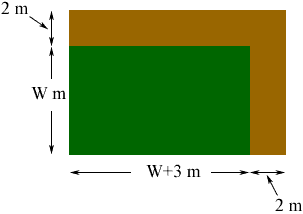Quandaries and Queries 1.  Increasing area of a field:  Julia's soybean field is 3m longer than it is wide.  To increase her production, she plans to increase her to increase both the length and width by 2m.  If the new field is 46m2(2nd power) larger than the old field, then what are the dimensions of the old field?   2.  Width of a Football Field:  If the perimeter of a football field in the NFL including the end zones is 1040 ft and the field is 120 yd long, then what is the width of the field in feet? 3. Fencing dog pens Clint is constructing two adjacent rectangular dog pens. Each pen will be three times as long as it is wide, and the pens will share a common long side. If Clint has 65 ft of fencing, what are the dimensions of each pen?   Adrian, Parent, and Secondary (10-12). Hi Adrian, All three of these problems involve drawing a diagram, labeling the appropriate quantities, using the diagram to develop an equation and then solving the equation. I'll do the first on and then your child can do the other two. The diagram for the first problem includes the original field as well as the extended field.I called the width of Julia's soybean field W meters and then its length is W + 3 meters since it is 3 meters longer than it is wide. She is going to add an additional 2 meters in length and an additional 2 meters in width so I drew these strips also. The area of the original field is W(W + 3) square meters s and the area of the extended field is (W + 2)(W + 5) square meters. You know that the extended field is 46 square meters larger than the original field so (W + 2)(W + 5) = W(W + 3) + 46 Solve for W. Penny Go to Math Central